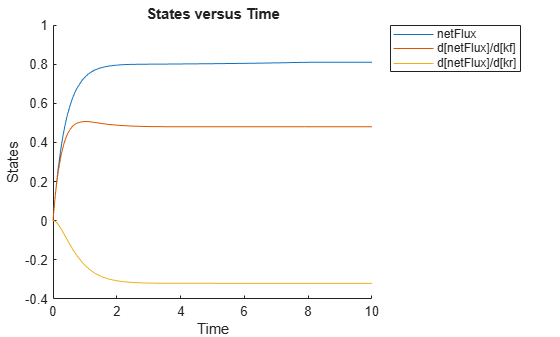# simbio.complexstep.abs

`abs` function for SimBiology local sensitivity analysis

## Syntax

``M = simbio.complexstep.abs(X)``

## Description

example

````M = simbio.complexstep.abs(X)` returns `M`, where `M = abs(real(X)) + 1i*imag(X)`. `simbio.complexstep.abs` is equivalent to `abs` when the input is real.When you have a SimBiology model with a custom function that calls `abs` and you are performing local sensitivity analysis on the model, replace `abs` with `simbio.complexstep.abs` in your custom function. You do not need to update SimBiology expressions (such as reaction rates or rules) that directly call `abs`. SimBiology automatically replaces `abs` with `simbio.complexstep.abs` whenever: You calculate local sensitivities using `sbiosimulate`, a `SimFunctionSensitivity` object, or the Calculate Sensitivities program.`sbiofit` or `fitproblem` uses local sensitivity analysis to determine the gradients of the objective function during parameter estimation. `simbio.complexstep.abs` is not differentiable when the real part of the input is 0 . For more information, see Local Sensitivity Analysis (LSA).```

## Examples

collapse all

This example shows how to use replacements for `abs`, `min`, and `max` in some custom functions so that the model becomes compatible with local sensitivity analysis (LSA). The replacement functions are `simbio.complexstep.abs`, `simbio.complexstep.min`, and `simbio.complexstep.max`. Specifically, one of the custom functions used by this example computes the net amount of a drug species moved between two compartments. The other custom function sets the thresholds for the forward and reverse reaction fluxes.

Create a model.

```model = sbiomodel("model"); c1 = addcompartment(model,"c1"); c2 = addcompartment(model,"c2"); s1 = addspecies(c1,"Drug",2); s2 = addspecies(c2,"Drug"); netFlux = addspecies(c1,"netFlux"); reaction = addreaction(model,"c1.Drug <-> c2.Drug"); kf = addparameter(model,"kf",1.0); kr = addparameter(model,"kr",1.5); fluxMin = addparameter(model,"fluxMin",0.1); fluxMax = addparameter(model,"fluxMax",10);```

Define the net amount of drug species moved between two compartments using a custom function `calculateNetFlux` based on the constrained forward and reverse reaction fluxes, which are defined later.

`rule = addrule(model,"netFlux = calculateNetFlux(boundedForwardFlux,boundedReverseFlux)","rate");`

calculateNetFlux uses `simbio.complexstep.abs`, and the function is already saved in the provided file named calculateNetFlux`.m`.

`type calculateNetFlux.m`
```function netFlux = calculateNetAmount(forwardFlux,reverseFlux) netFlux = simbio.complexstep.abs(forwardFlux-reverseFlux); end ```

Define the forward and reverse fluxes of the reaction. Set the thresholds on the fluxes using the `imposeBounds` custom function.

```boundedForwardFlux = addparameter(model,"boundedForwardFlux","Constant",false); boundedReverseFlux = addparameter(model,"boundedReverseFlux","Constant",false); forwardFlux = addparameter(model,"forwardFlux","Constant",false); reverseFlux = addparameter(model,"reverseFlux","Constant",false); forwardFlux = addrule(model,"forwardFlux = kf*c1.Drug","repeatedAssignment"); reverseFlux = addrule(model,"reverseFlux = kr*c2.Drug","repeatedAssignment"); boundedForwardFlux = addrule(model,"boundedForwardFlux = imposeBounds(forwardFlux,fluxMin,fluxMax)","repeatedAssignment"); boundedReverseFlux = addrule(model,"boundedReverseFlux = imposeBounds(reverseFlux,fluxMin,fluxMax)","repeatedAssignment"); reaction.ReactionRate = "boundedForwardFlux - boundedReverseFlux";```

`imposeBounds` uses `simbio.complexstep.min` and `simbio.complexstep.max` to set the lower and upper limits for the reaction flux.

`type imposeBounds.m`
```function boundedFlux = imposeBounds(fluxInput,fluxMin,fluxMax) fm = simbio.complexstep.max(fluxMin,fluxInput); boundedFlux = simbio.complexstep.min(fluxMax,fm); end ```

Enable local sensitivity analysis.

```configset = getconfigset(model); configset.RuntimeOptions.StatesToLog = "netFlux"; configset.SolverOptions.SensitivityAnalysis = true; sensitivityOptions = configset.SensitivityAnalysisOptions; sensitivityOptions.Inputs = [kf,kr]; sensitivityOptions.Outputs = netFlux;```

Temporarily disable the warning about unsupported functions. The warning is safe to ignore.

```warnState = warning("off","SimBiology:senscsverify:UnsupportedFunction"); cleanupobj = onCleanup(@()warning(warnState));```

Simulate the model and perform sensitivity analysis.

```simdata = sbiosimulate(model); sbioplot(simdata);````delete(cleanupobj);`

## Input Arguments

collapse all

Input array, specified as a numeric scalar, vector, matrix, or multidimensional array.

Data Types: `double`
Complex Number Support: Yes

## Output Arguments

collapse all

Output array, returned as a numeric scalar, vector, matrix, or multidimensional array. The size of the output array is the same as the input array.

• For a complex number `x` with a nonnegative real part, `simbio.complexstep.abs(x) = x`.

• For a complex number `x` with a negative real part, `simbio.complexstep.abs(x) = -real(x) + 1i*imag(X)`.

 Martins, Joaquim, Ilan Kroo, and Juan Alonso. “An Automated Method for Sensitivity Analysis Using Complex Variables.” In 38th Aerospace Sciences Meeting and Exhibit. Reno, NV, U.S.A.: American Institute of Aeronautics and Astronautics, 2000. https://doi.org/10.2514/6.2000-689.